# chapter 1 Functions Exercise 1a Solutions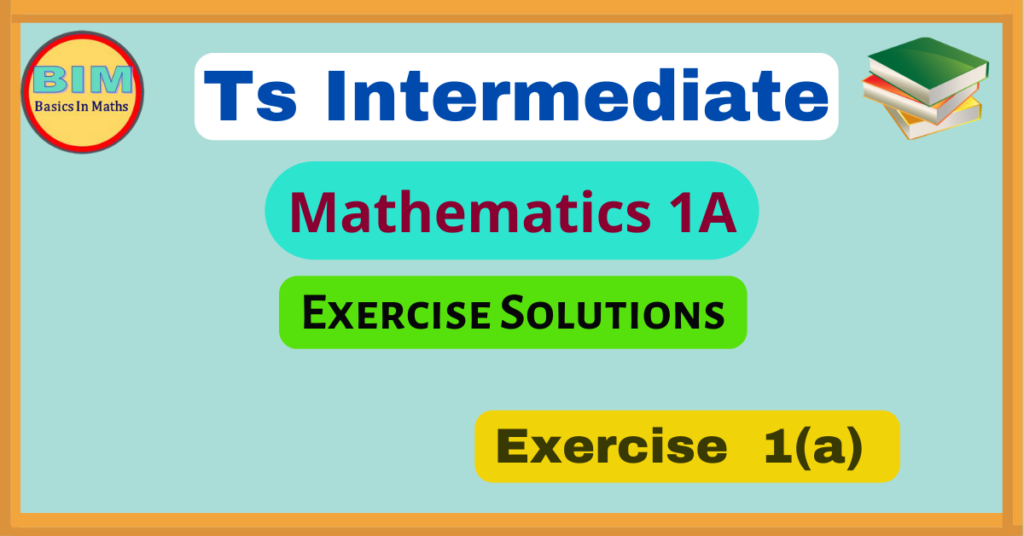## Functions Exercise 1a Solutions

Functions Exercise 1a

The famous mathematician ” Lejeune Dirichlet”  defined a function.

Function: A variable is a symbol which represents any one of a set of numbers, if two variables x and y so related that whenever a value is assigned to x there is autometically assigned by some rule or correspondence a value to y, then we say y is a function of x.

Chapter 1 Functions Exercise 1a Solutions for inter first year  students, prepared by Mathematics expert of www.basicsinmaths.com

### Exercise 1a

#### I.

##### 1. If the function f is defined bythen find the values of (i) f (3)    (ii) f (0)      (iii) f (– 1.5)       (iv) f (2) + f (– 2)       (v) f (– 5 )

Sol:

GivenDomain of f(x) is (– 3, ∞)

(i) f (3)

3 lies in the interval x > 1

⟹ f(x) = x + 2

f(3) = 3 + 2 = 5

∴ f (3) = 5

(ii) f (0)

0 lies in interval – 1 ≤ x ≤ 1

⟹f(x) = 2

∴ f (0) = 2

(iii) f (– 1.5)

– 1.5 lies in interval – 3 < x < – 1

⟹f(x) = x – 1

f (– 1.5) = – 1.5 – 1 = – 2.5

∴ f (– 1.5) = – 2.5

(iv) f (2) + f (– 2)

2 lies in the interval x > 1

⟹ f (x) = x + 2

f (3) = 2 + 2 = 4

f (2) = 4

– 2 lies in interval – 3 < x < – 1

⟹f(x) = x – 1

f (– 2) = – 2 – 1 = – 3

f (– 2) = – 2 – 1 = – 3

now f (2) + f (– 2) = 4 – 3 = 1

∴ f (2) + f (– 2) = 1

(v) f (– 5)

since domain of f(x) is (– 3, ∞)

f (– 5) is not defined

##### 2. If f: R – {0} ⟶ R is defined by f(x) =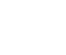, then show that f (x) + f (1/x) = 0

Sol:

Given f: R – {0} ⟶ R is defined by f(x) =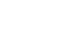f (1/x)  =Now

f (x) + f (1/x)  =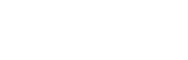∴ f (x) + f (1/x)  = 0

##### 3. If f: R ⟶ R is defined by f(x) =, then show that f (tan θ) = cos 2θ

Sol:

Given f: R ⟶ R is defined by f(x) =f (tan θ) == cos 2θ##### 4. If f: R – {±1} ⟶ R is defined by f(x) =, then show that f= 2 f (x)

Sol:

Given f: R – {±1} ⟶ R is defined by f(x) =##### 5. If A = {– 2, – 1, 0, 1, 2} and f: A ⟶ B is a surjection (onto function) defined by f(x) = x2 + x + 1, then find B

Sol:

Given A = {– 2, – 1, 0, 1, 2} and   f: A ⟶ B is a surjection defined by f(x) = x2 + x + 1

f(– 2) = (–2)2 + (–2) + 1

= 4 – 2 + 1 = 3

f(– 1) = (–1)2 + (–1) + 1

= 1 – 1 + 1 = 1

f(0) = (0)2 + (0) + 1

= 0 + 0 + 1 = 1

f(1) = (1)2 + (1) + 1

=1 +1 + 1 = 3

f( 2) = (2)2 + (2) + 1

= 4 + 2 + 1 = 4

∴ B = {1, 3, 7}

TS 10th class maths concept (E/M)

##### 6. If A = {1, 2, 3,4} and f: A ⟶ B is a surjection defined by f(x) =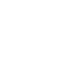, then find range of f.

Sol:

Given A = {1, 2, 3,4} and f: A ⟶ B is a surjection defined by f(x) =##### 7. If f (x + y) = f (xy) ∀ x, y ∈ R, then prove that f is a constant function

Sol:

Given f (x + y) = f (xy) ∀ x, y ∈ R

Let x = 0 and y = 0

f (0 + 0) = f (0 × 0) = f (0)

f (1) = f (0 + 1)

= f (0 × 1)

= f (0)

f (2) = f (1 + 1)

= f (1 × 1)

= f (1)

= f (0)

f (3) = f (1 + 2)

= f (1 × 2)

= f (2)

= f(0)

Similarly, f(4) = 0

f(5) = 0

and so on.

∴ f is a constant function

II.

##### 1. If A = {x/ – 1 ≤ x ≤ 1}, f(x) = x2, g(x) = x3, which of the following are surjections?

(i) f: A ⟶ A                 (ii) g: A ⟶ A

Sol:

###### (i) Given A = {x/ – 1 ≤ x ≤ 1}, f(x) = x2

A = {– 1, 0, 1}; f: A ⟶ A

f (x) = x2

f (– 1) = (– 1)2 = 1

f (0) = (0)2 = 0

f (1) = (1)2 = 1

∵ range is not equal to co domain of f

f is nor a surjection

###### (ii) A = {x/ – 1 ≤ x ≤ 1}, g (x) = x3

A = {– 1, 0, 1}; g: A ⟶ A

g (x) = x3

g (– 1) = (– 1)3 = – 1

g (0) = (0)3 = 0

g (1) = (1)3 = 1

∵ range is equal to co domain of f

f is a surjection

#### 2. Which if the following are injection, surjection or bijection? Justify your answer

##### (i) f: R ⟶ R defined by f(x) =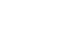let x1, x2 ∈ R

f(x1) = f(x2)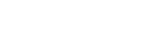2x1 + 1 = 2x2 + 1

2x1 = 2x2

x1 = x2

x1, x2 ∈ R, f(x1) = f(x2) ⟹ x1 = x2

∴ f is an injection

Let y = f(x)

y =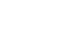3y = 2x + 1

3y – 1 = 2x

⟹ x =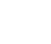∈ R

Now∴ f is surjection

f is injection and surjection

∴ f is a bijection

##### (ii) f: R ⟶ (0, ∞) defined by f(x) = 2x

let x1, x2 ∈ R

f(x1) = f(x2)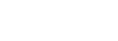x1 = x2

x1, x2 ∈ R, f(x1) = f(x2) ⟹ x1 = x2

∴ f is an injection

Let y = f(x)

y = 2x

x =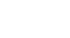∈ (0, ∞)

Now f(x) = 2x

=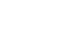= y

∴ f is surjection

f is injection and surjection

∴ f is a bijection

##### (iii) f: (0, ∞) ⟶ R defined by f(x) =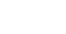let x1, x2 ∈ (0, ∞)

f(x1) = f(x2)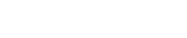x1 = x2

x1, x2 ∈ (0, ∞), f(x1) = f(x2) ⟹ x1 = x2

∴ f is an injection

Let y = f(x)

y =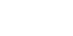x = ey ∈ (0, ∞)

Now f(x) ==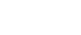= y

∴ f is surjection

f is injection and surjection

∴ f is a bijection

##### (iv) f: [0, ∞) ⟶ [0, ∞) defined by f(x) = x2

let x1, x2 ∈ [0, ∞)

f(x1) = f(x2)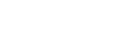x1 = x2 [∵ x1, x2 ∈ [0, ∞)]

x1, x2 ∈ [0, ∞), f(x1) = f(x2) ⟹ x1 = x2

∴ f is an injection

Let y = f(x)

y = x2

x =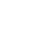∈ [0, ∞)

Now f(x) = x2

=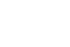= y

∴ f is surjection

f is injection and surjection

∴ f is a bijection

##### (v) f: R ⟶ [0, ∞) defined by f(x) = x2

let x1, x2 ∈ R

f(x1) = f(x2)x1 = ± x2 [∵ x1, x2 ∈ R]

x1, x2 ∈ [0, ∞), f(x1) = f(x2) ⟹ x1 ≠ x2

∴ f is not an injection

Let y = f(x)

y = x2

x =∈ R

Now f(x) = x2

== y

∴ f is surjection

f is not an injection but surjection

∴ f is not a bijection

##### (vi) f: R ⟶ R defined by f(x) = x2

let x1, x2 ∈ R

f(x1) = f(x2)

x1 = ± x2 [∵ x1, x2 ∈ R]

x1, x2 ∈ [0, ∞), f(x1) = f(x2) ⟹ x1 ≠ x2

∴ f is not an injection

f (1) = 12 = 1

f (– 1) = (–1)2 = 1

here ‘– 1’ has no pre image

∴ f is not a surjection

f is not an injection and not surjection

∴ f is not a bijection

Ts Inter Maths IA Concept

hai

#### 3. Is g = {(1, 1), (2, 3), (3, 5), (4, 7)} is a function from A = {1, 2, 3, 4} to B = {1, 3, 5, 7}? If this is given by the formula g(x) = ax + b, then find a and b

Sol:

Given A = {1, 2, 3, 4}, B = {1, 3, 5, 7} and g = {(1, 1), (2, 3), (3, 5), (4, 7)}

g (1) = 1; g(2) = 3; g(3) = 5 ; g(4) = 7

here, every element of set A has a unique image in set B

∴ g: A ⟶ B is a function

And also given g(x) = ax + b

g (1) = 1

⟹ a (1) + b = 1

a + b = 1

b = 1 – a _______________   (1)

g (2) = 3

a (2) + b = 3

2a + b = 3

2a + 1 – a = 3 (from (1))

a+ 1 = 3

a = 2

b = 1 – 2

b = – 1

∴ a = 2, b = – 1

#### 4. If the function f: R ⟶ R defined by f(x) =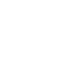, then show that f (x + y) + f (x – y) = 2 f (x) f (y).

Sol:

Given the function f: R ⟶ R defined by f(x) == 2 f (x) f (y)

5. If the function f: R ⟶ R defined by f(x) =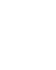, then show that f (1 – x) = 1 – f (x)

and hence reduce the value ofSol:

Given the function f: R ⟶ R defined by f(x) =∴ f (1 – x) = 1 – f(x)

#### 6. If the function f: {– 1, 1} ⟶ {0, 2}, defined by f(x) = ax + b is a surjection, then find a and b

Sol:

Given the function f: {– 1, 1} ⟶ {0, 2}, defined by f(x) = ax + b is a surjection

Case(i)If f(– 1) = 0 and f(1) = 2

a (– 1) + b = 0

– a + b = 0

b = a ————(1)

and

a (1) + b = 2

a + b = 2

a + a = 2         [ from (1)]

2a = 2

a = 1

b = 1

Case (ii)If f(– 1) = 2 and f(1) = 0

a (– 1) + b = 2

– a + b =

b = 2 + a ————(2)

and

a (1) + b = 0

a + b = 0

a + 2 + a = 0         [ from (2)]

2a + 2 = 0

2a = – 2

a = – 1

b = 1

From Case(i) and  Case (ii) a = ±1 and b = 1

##### 7. If f(x) = cos (log x), then show that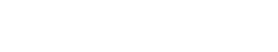= 0

Sol

Given f(x) = cos (log x)#####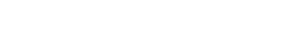=

cos (log x) cos (log y) –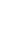[cos (log x) cos (log y) – sin (log x) sin (log x)

+ cos (log x) cos (log y) + sin (log x) sin (log x)]

= cos (log x) cos (log y) –[2cos (log x) cos (log y)]

= cos (log x) cos (log y) – cos (log x) cos (log y)

∴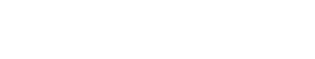= 0

##### Visit my Youtube Channel: Click on Below Logo